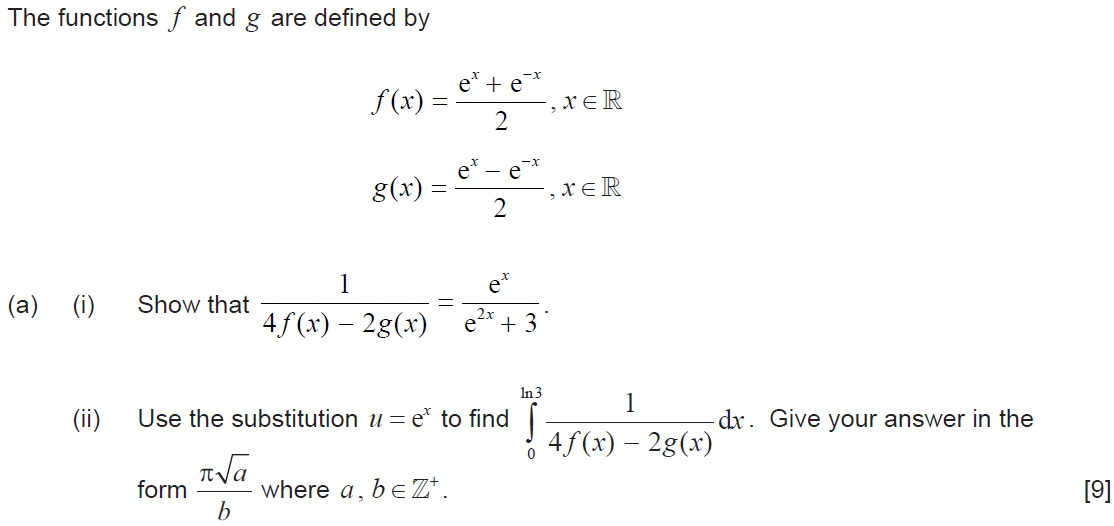# IBDP Past Year Exam Questions – Techniques for Integration

1. [M08/P2/TZ1]

By using an appropriate substitution find

2. [M98/P1]

Find     .

3. [N95/P1]

Find  .

4. [M11/P2/TZ2]

Using integration by parts, show that  . [6 marks]

5. [N12/P2/TZ2]

By using the substitution  , find  $\int \frac{{x}^{3}}{\sqrt{1–{x}^{2}}}dx$ .    [7 marks]

6. [M13/P1/TZ1]

The function  $f$ is defined by  $f\left(x\right)=\frac{1}{4{x}^{2}–4x+5}$ . By using a suitable substitution show that  .

7. [N13/P2/TZ0]

By using the substitution  , show that

$\int \frac{dx}{{x}^{2}\sqrt{{x}^{2}+4}}=\frac{–\sqrt{{x}^{2}+4}}{4x}+C$         [7 marks]

8. [N14/P1/TZ0]

By using the substitution  $u=1+\sqrt{x}$ , find  .   [6 marks]

9. [M16/P2/TZ2]10. [N17/P2/TZ0]

By using the substitution  , show that  $\int \frac{dx}{x\sqrt{{x}^{4}–4}}=\frac{1}{4}arc\mathrm{cos}\left(\frac{2}{{x}^{2}}\right)+c$ .     [7 marks]